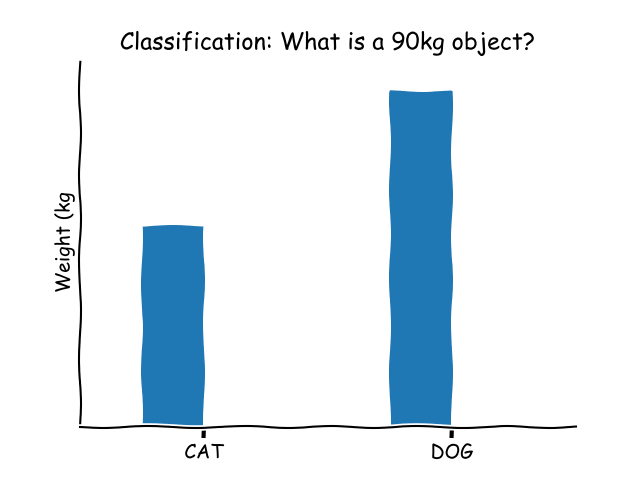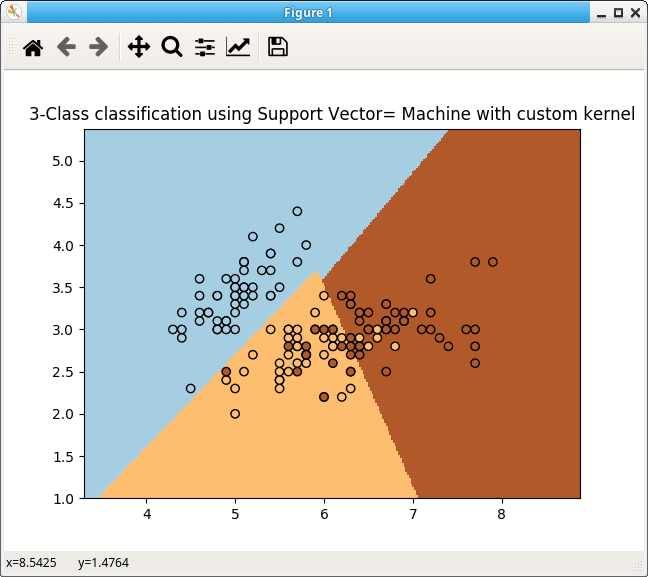Support Vector Machine (SVM) Algorithms were invented by Vladimir Vapnik and Alexey Chervonenkis in 1963 and are capable of it. But, it wasn’t until the mid-90’s that a community grown around them.

Support Vector Machines (SVM) can be described as family of algorithms that learns from data by creating models that maximize their margin of error. That’s they work, choosing a model that maximizes the error margin of a training set.

### Classification

They can be used for classification problems.SVM is a discriminative classifier formally defined by a separating hyperplane. Once given labeled training data, the algorithm outputs an optimal hyperplane which categorizes new examples.

## Why?

Support Vector Machines are user-friendly. Why?

• Are easy to understand and code.
• It has a method for calibrating the output to yield probabilities.
• It has a method to apply to find unusual items in a training set.
• It has a simple method to convert a multi-class problem into a series of faster-to-solve two-class.

These are some of its applications.

• Text and hypertext categorization, as their application can significantly reduce the need for labeled training instances.
• Classification of images can be performed. It achieves high search accuracy than traditional query refinement schemes.
• Hand-written characters can be recognized using it.
• Sciences classification, for example, the proteins can be classified with high accuracy.

## How’s the data preparation for Support Vector Machine?

It is either Numeral Inputs or Binary Classification.

Numerical Inputs when Support Vector Machine assumes that your inputs are numeric. If you have categorical inputs you may need to convert them to binary dummy variables.

Binary Classification when the Support Vector Machine is intended for binary classification problems.

## SVM

Any Support Vector Machine needs input data, because it is a supervised learning algorithm. It needs training data before it can make predictions.The numeric input variables (let’s imagine you have two) in the data form an n-dimensional space (if you have two, then it’s a two-dimensional space).

You can create and train SVM like this:

Then you can make predictions

There’s a lot to learn on Support Vector Machines, but this summary can give you an idea of what these algorithms are.